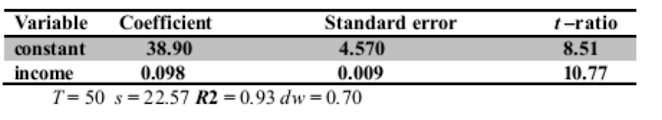# Identify Whether The Statements From (A)-(C) Ate True Or False, And Explain The Reason Intensely:

1. What are the important reasons econometricians used lag values of a time series variable?

2. Identify whether the statements from (a)-(c) ate true or false, and explain the reason intensely.

• One of the assumptions that need to hold for the process tyt} to be weakly stationary is that co v(yt, yt-k) is constant over time and depends on both t and k.
• A white noise process is a non-stationary process for which all autocorrelations are equal to zero.
• If our series are non-stationary, it is safe to use OLS as our estimation method.

3. Suppose we have the following model: Y,= a+ flXi+ U,

where the explanatory variable X, is strictly exogenous, and the residual U, is serially correlated.

• Why is serial correlation often present in time series data?
• Why is the presence of serial correlation in the residual a problem?

Suppose we can express serial correlation in U, in the equation above as follows:

U, = PU,•, +e,

State the null hypothesis for testing serial correlation in the equation.

4. Consider the following time series model: Y, = ti Vt.] + Ut

where it follows a white noise process.

a. What is the condition we need to impose on t, in order for the series yet to be weakly stationary? Why?

5. Suppose aggregate savings explained from aggregate income for Ethiopia is estimated by OLS over the years 1946-1995(T= 50). The results are given below: S, = a + Mc c